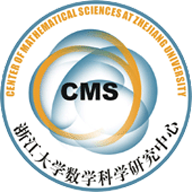2019-05-21 来源：数学科学研究中心

The first part of the talk focuses on robust covariance matrix estimation. To deal with modern complex data sets, not only do we need estimation procedures to take advantage of the structural assumptions of the covariance matrix, it is also important to design methods that are resistant to arbitrary source of outliers. To this end, we define a new concept called matrix depth and propose to maximize the empirical matrix depth function to obtain a robust covariance matrix estimator. Under Huber's contamination model, the proposed estimator is shown to achieve minimax optimal rate under the spectral norm loss for estimating covariance/scatter matrices with various structures such as bandedness and sparsity.

We then revisit the classical nonparametric density estimation under Huber's contamination model and consider various $\ell_p$ losses ($1\leq p< \infty$). We carefully study the effect of contamination on estimation through the following model indices: contamination proportion, smoothness of target density, smoothness of contamination density, and the choice of the loss function.

In the end, following the above framework, we further establish a general decision theory for robust statistics under Huber's contamination model. When the loss is equivalent to the total variation distance, we propose a solution using Scheff{\'e} estimate to a robust two-point testing problem that leads to the construction of robust estimators adaptive to the proportion of contamination. Applying the general theory, we construct robust estimators for nonparametric density estimation, sparse linear regression and low-rank trace regression. We show that these new estimators achieve the minimax rate with optimal dependence on the contamination proportion. This testing procedure, Scheff{\'e} estimate, also enjoys an optimal rate in the exponent of the testing error, which may be of independent interest.abstract.pdf